# Scalar A scalar quantity has magnitude size only

• Slides: 17Scalar: A scalar quantity has magnitude (size) only, but no direction. Examples include: time, mass, distance and speed. Vector: A vector quantity has both magnitude and direction. Examples include: displacement, velocity and force.The position of an object is the separation between that object and a reference point. (which is usually “zero” on the scale) The position of car B is 1. 0 m to the left of the reference. The position of car A is 8. 0 m to the right of the reference. Since we stated the direction, position is a VECTOR quantity.Distance, on the other hand, needs no frame of reference. You measure the distance between two objects by measuring their separation. Car A is 9. 0 m from car B no matter where you put the reference point. Since we did not state the direction, distance is a SCALAR quantity.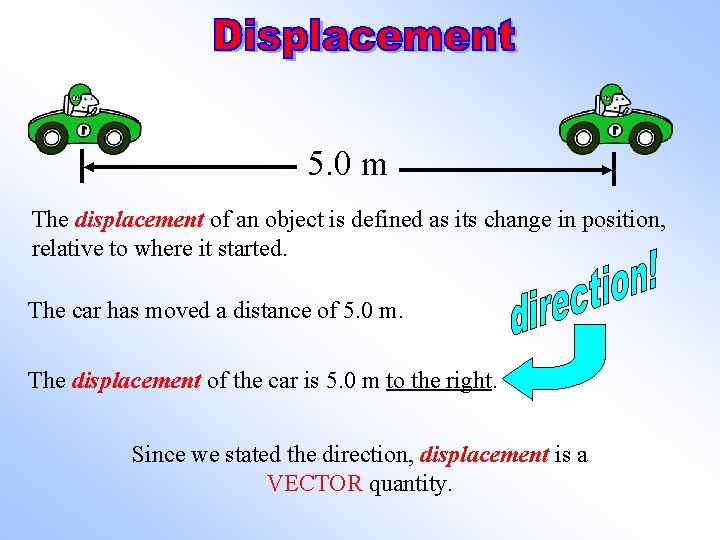5. 0 m The displacement of an object is defined as its change in position, relative to where it started. The car has moved a distance of 5. 0 m. The displacement of the car is 5. 0 m to the right. Since we stated the direction, displacement is a VECTOR quantity.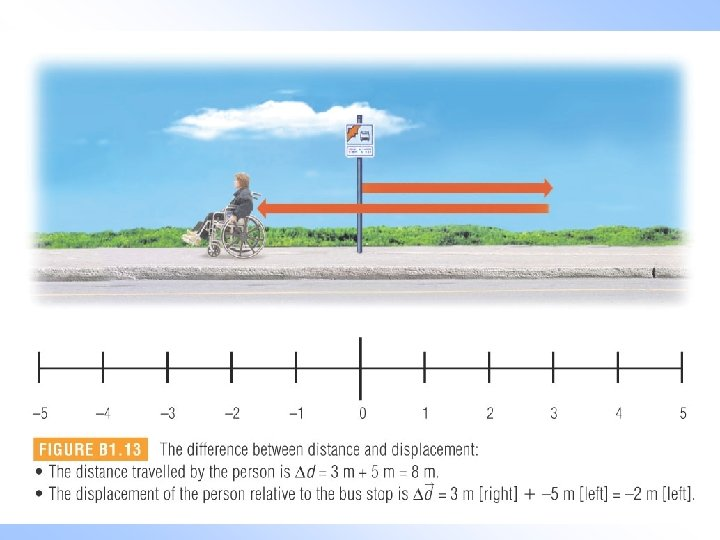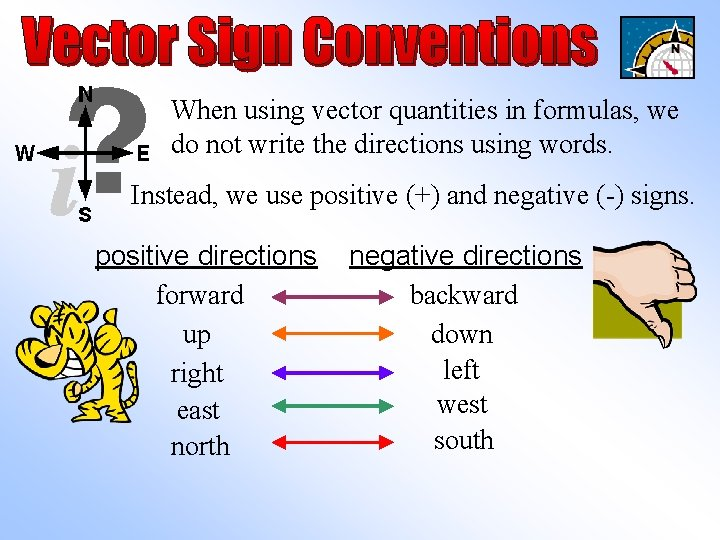When using vector quantities in formulas, we do not write the directions using words. Instead, we use positive (+) and negative (-) signs. positive directions forward up right east north negative directions backward down left west southX-Axis Method How to use the X-axis Method: Up (90 o) Left (180 o) 40 o Right (0 o) Read the grid from 0 o counterclockwise and include direction. • Up and right are positive 5 m • Down and left are negative Down (270 o) • Directions between axis lines are given only in degrees. For Example: Vector A is positioned at 5 m (220 o) See page 139, example problem B 1. 4 for more examples.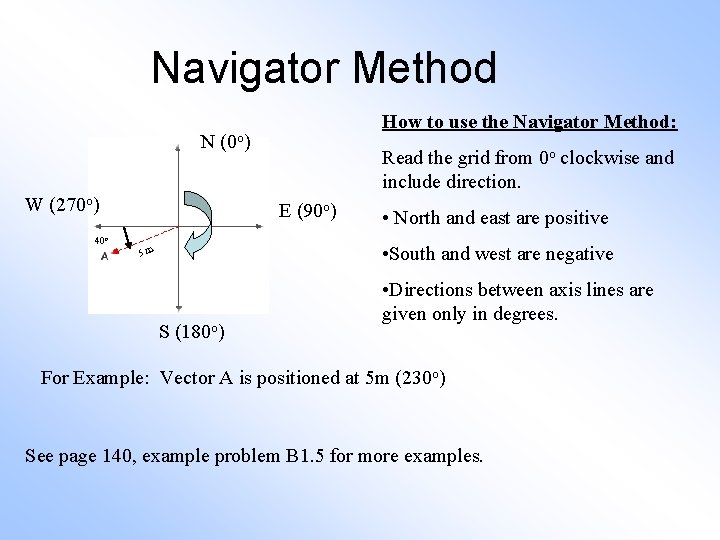Navigator Method How to use the Navigator Method: N (0 o) W (270 o) 40 o Read the grid from 0 o clockwise and include direction. E (90 o) • North and east are positive • South and west are negative 5 m S (180 o) • Directions between axis lines are given only in degrees. For Example: Vector A is positioned at 5 m (230 o) See page 140, example problem B 1. 5 for more examples.Navigator II Method (‘of’ Method)t = 1. 2 s 5. 0 m The car has a displacement of 5. 0 m to the right in 1. 2 s. The average velocity of the car is defined as a change in position during a time interval. It is called an average velocity because it does not take into account speeding up and slowing down. = average velocity (m/s) = displacement (m) Δt = time (s) We use the arrows “→” to indicate vector quantities.t = 1. 2 s 5. 0 m Remember to state the direction with vector quantities! = 4. 2 m/s to the right Since we stated the direction, average velocity is a VECTOR quantity.Practice Problems p. 141 8) A student walks 10. 0 m [E] in 7. 00 s. Then he walks another 12. 0 m [E] in 8. 00 s. Determine: a) the displacement of the student in 15. 00 s b) the average velocity of the student. 22. 0 m [E] 1. 47 m/s [E] 9) A boat travels at a velocity of 8. 00 m/s [N] for 14. 0 s. What is the displacement of the boat? 112 m [N] 10) An airplane flying at a velocity of 900 km/h [W] travels 400 km west. How long will the plane be in flight? 0. 444 h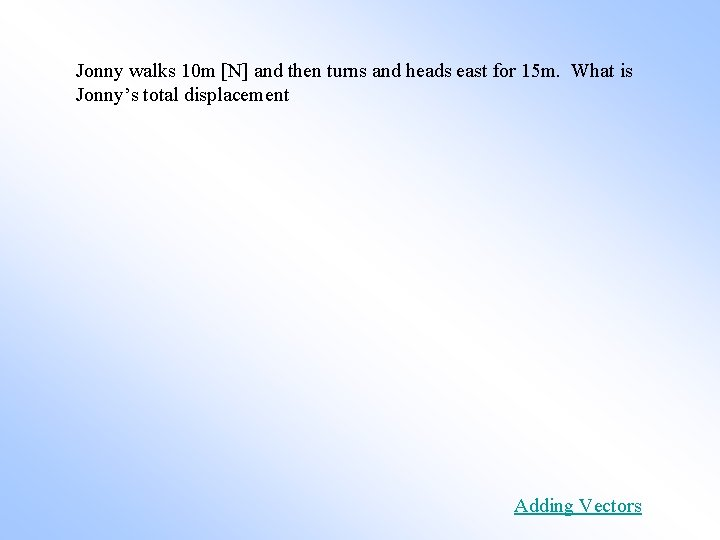Jonny walks 10 m [N] and then turns and heads east for 15 m. What is Jonny’s total displacement Adding VectorsThe only difference between distance-time graphs and position-time graphs is that direction is included. This means that the slope is equal to the velocity.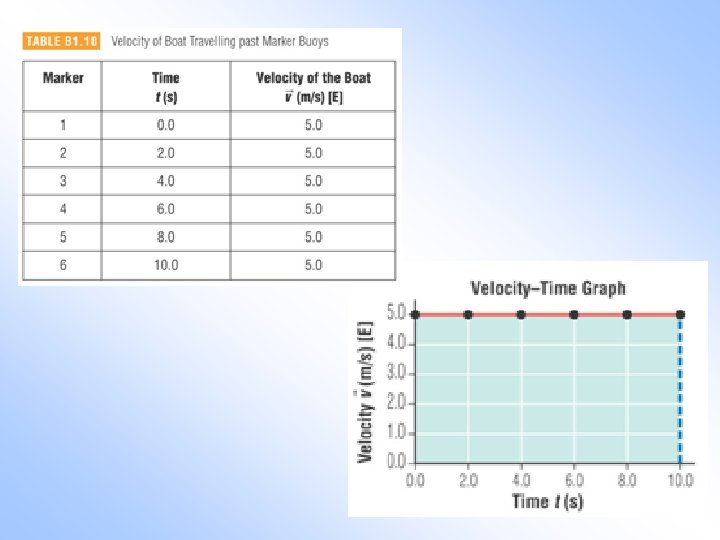ü read pages 137 – 144 ü Line master 3 – Graphical analysis if uniform motion (average velocity) ü B 1. 1 Check and Reflect page 145 #’s 1 -7